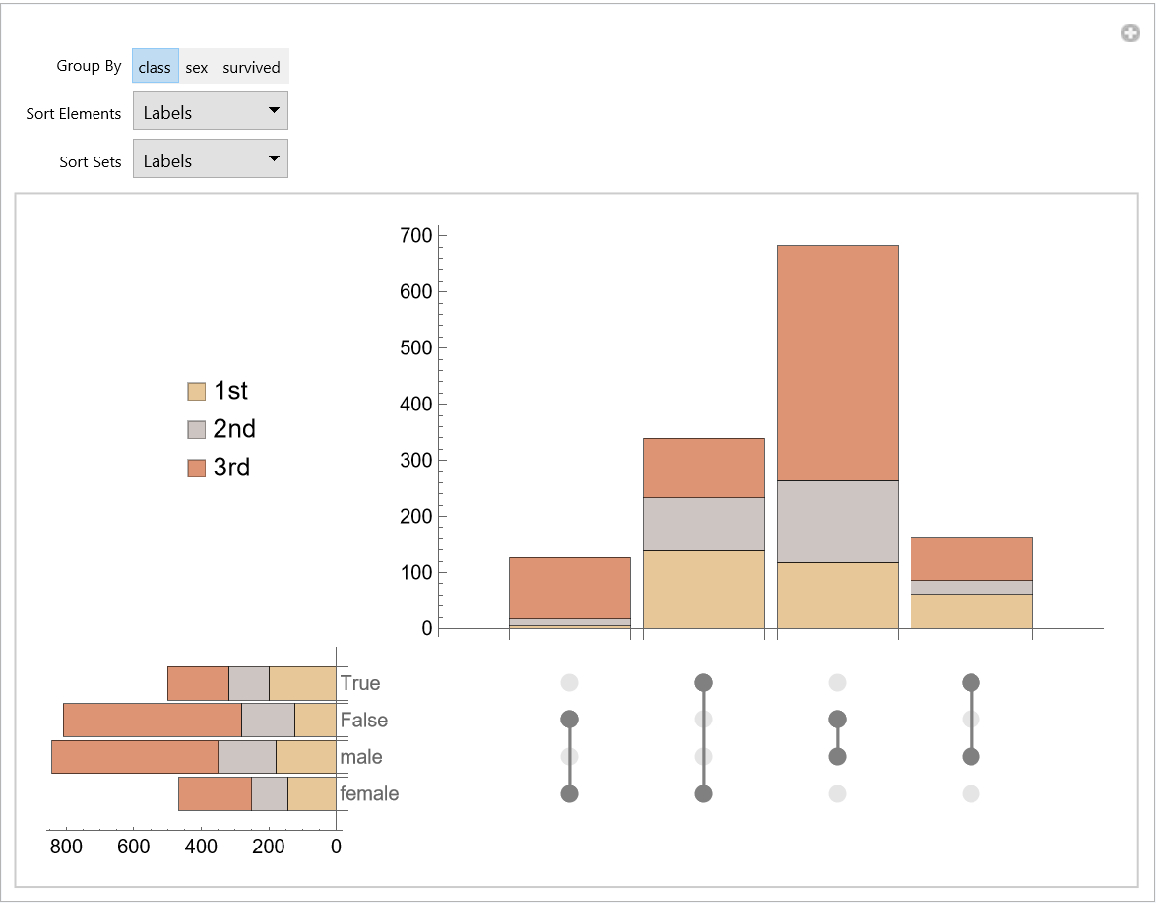Function Repository Resource:

UpSetChart

Visualize the intersections and aggregates of intersections of tuples of intersecting sets

Contributed by: Edmund B Robinson
 ResourceFunction["UpSetChart"][{tuple1,tuple2,…}] charts the list of related tuples {tuple1,tuple2,…}. ResourceFunction["UpSetChart"][{{tuple1,1,tuple1,2,…},{tuple2,1,tuple2,2,…},…}] charts the list of sublists of related tuples.

Details and Options

ResourceFunction["UpSetChart"] visualises tuples of intersecting sets.
ResourceFunction["UpSetChart"] has three sections:
 Set Menu Chart Lower Left BarChart of the cardinality of each element in the lists of tuples Cardinality Chart Upper Right BarChart of the cardinality of each subset of the lists of tuples Combination Matrix Plot Lower Right Plot of the subsets in the list of tuples
ResourceFunction["UpSetChart"] takes the following options:
 {1/GoldenRatio,1/GoldenRatio} aspect ratios used for the set menu and cardinarity sub-charts style used for placeholders in combination matrix sub-plot list of labels used for the legend for the set menu sub-chart list of styles used for the set menu and cardinality sub-charts {{20,2},{15,2}} image padding of the combination matrix sub-plot { , } size of the combination matrix sub-plot Graphics[Disk[{0,0},Scaled[0.025]]] markers used for the combination matrix sub-plot style used for the combination matrix sub-plot theme applied to all sub-plots and sub-charts "SortingFunctions" sort functions applied to the elements of the set menu and cardinality sub-charts
For the AspectRatio option {spec1,spec2}, spec1 is applied to the set menu sub-chart and spec2 to the cardinality sub-chart.
For the ImagePadding option {specx,specy}, specx is applied to combination matrix sub-plot and cardinality sub-chart, while specy is applied to the combination matrix sub-plot and set menu sub-chart.
The functions in the "SortingFunctions" option {f1,f2}take an association of counts:
 f 1 set menu sub-chart Keys are elements of the sets and Values are lists of counts in each set of tuples f 2 cardinality sub-chart Keys are the subsets of the tuples and Values are lists of counts in each set of tuples

Examples

Basic Examples (2)

Simulate data for a survey of 30 animal lovers for the types of pets they have:

 In:=Out=View relationships:

 In:=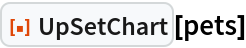Out=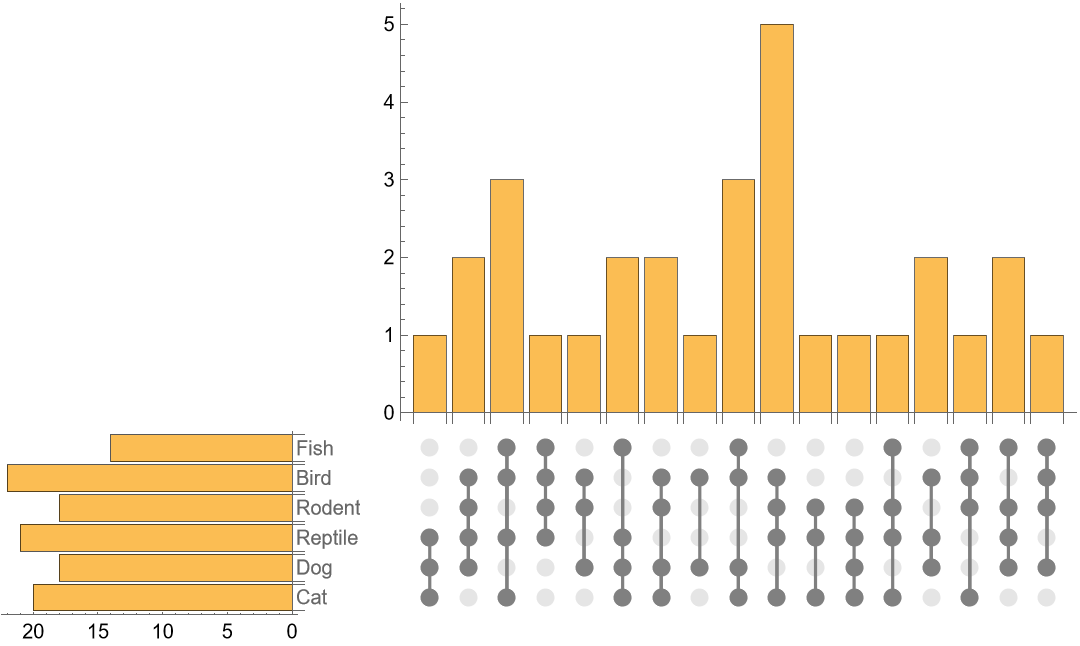Simulate data for a survey of 15 homes in 2 cities for the types of vehicles they own:

 In:=Out=View relationships of both categories:

 In:=Out=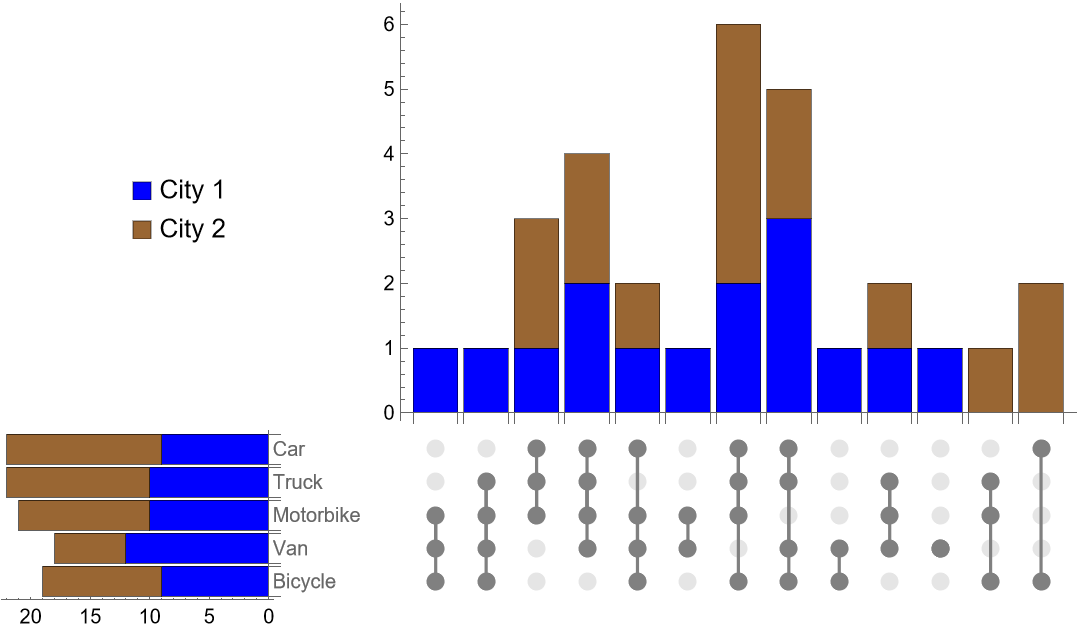Options (21)

AspectRatio (2)

Set menu and cardinality sub-charts are sized to the combination plot. However their size can be adjusted with AspectRatio. Use default sizes for a fixed sized combination plot:

 In:=Out=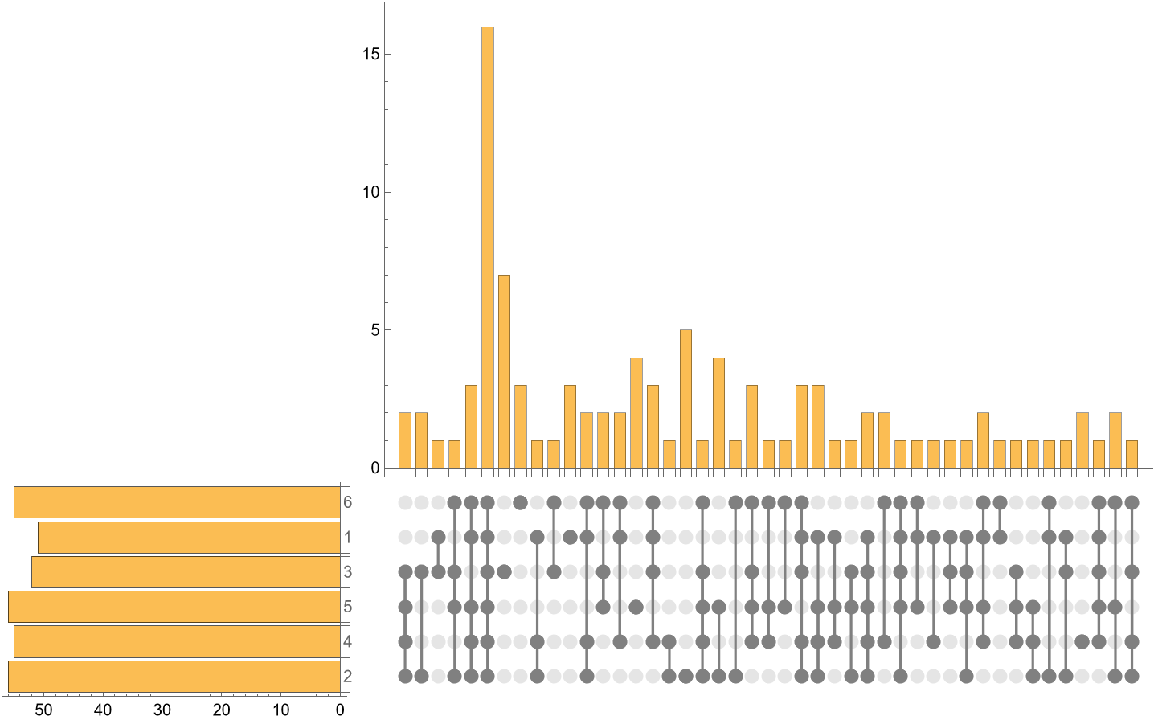Supply AspectRatio specification for set menu and cardinality sub-charts to adjust their size:

 In:=Out=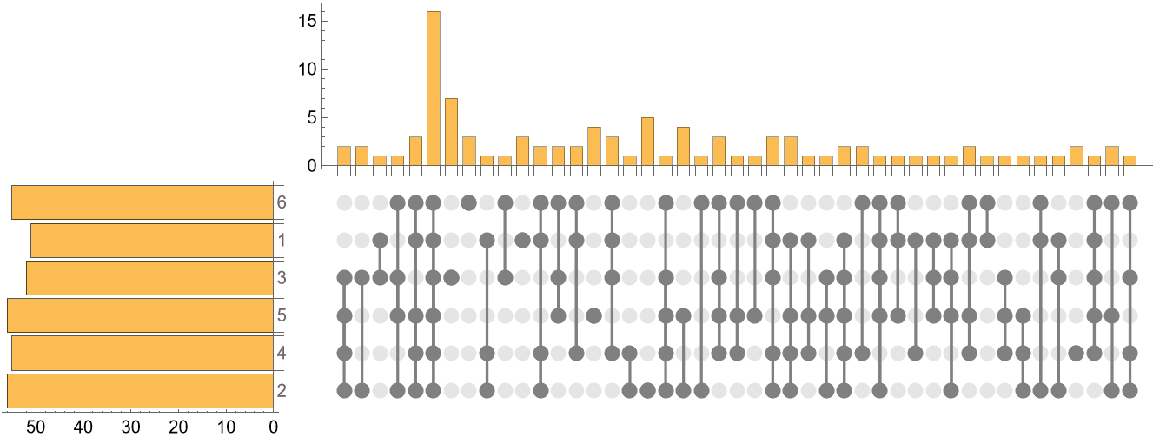Background (1)

Supply a style for the placeholders of the combination matrix sub-plot:

 In:=Out=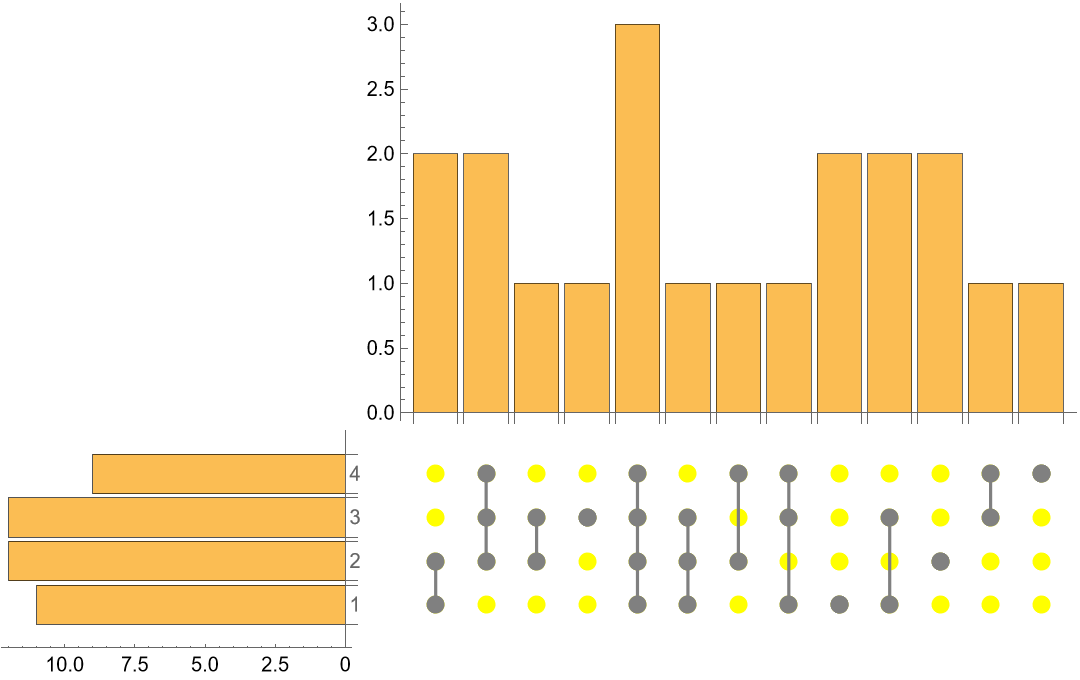ChartLegends (2)

Create example data:

 In:=Supply a list of labels and a list of styles:

 In:=Out=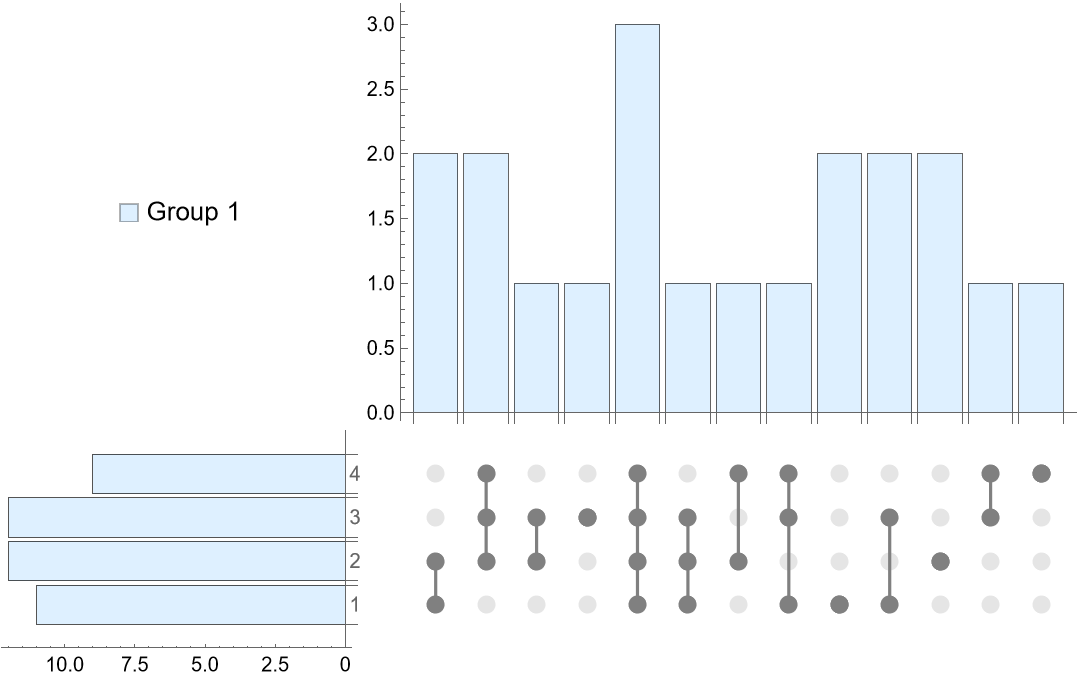ChartStyle (2)

Create example data:

 In:=Supply a list of styles:

 In:=Out=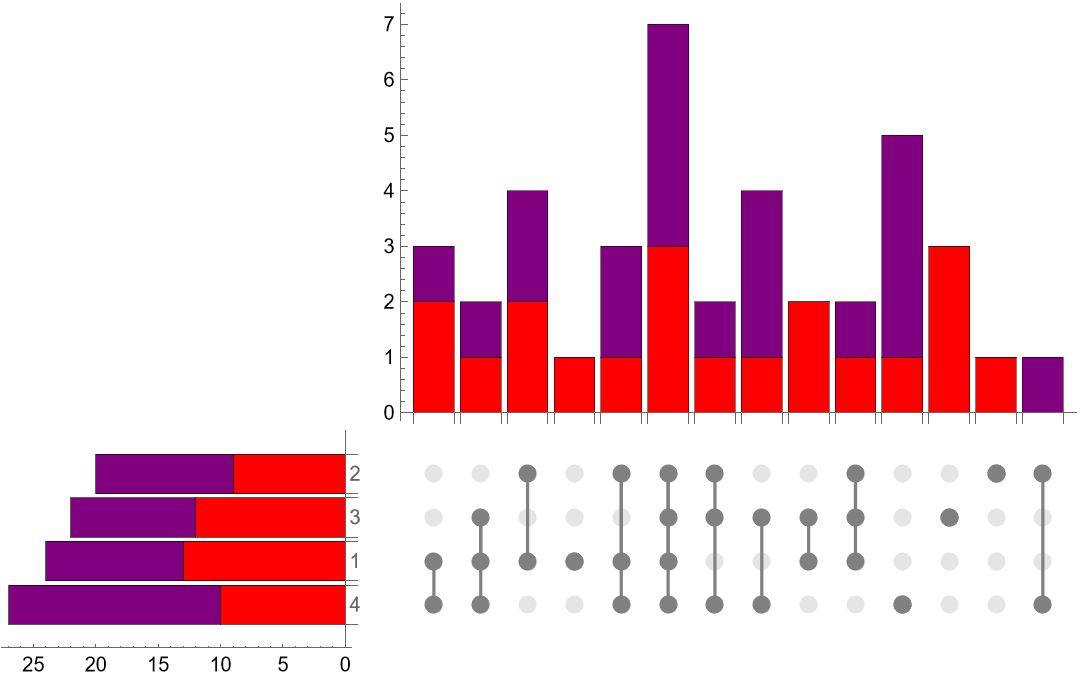Set menu and cardinality sub-charts image padding uses the combination plot's image padding. Default image padding may be too narrow to fit set menu or cardinality sub-chart axis labels:

 In:=Out=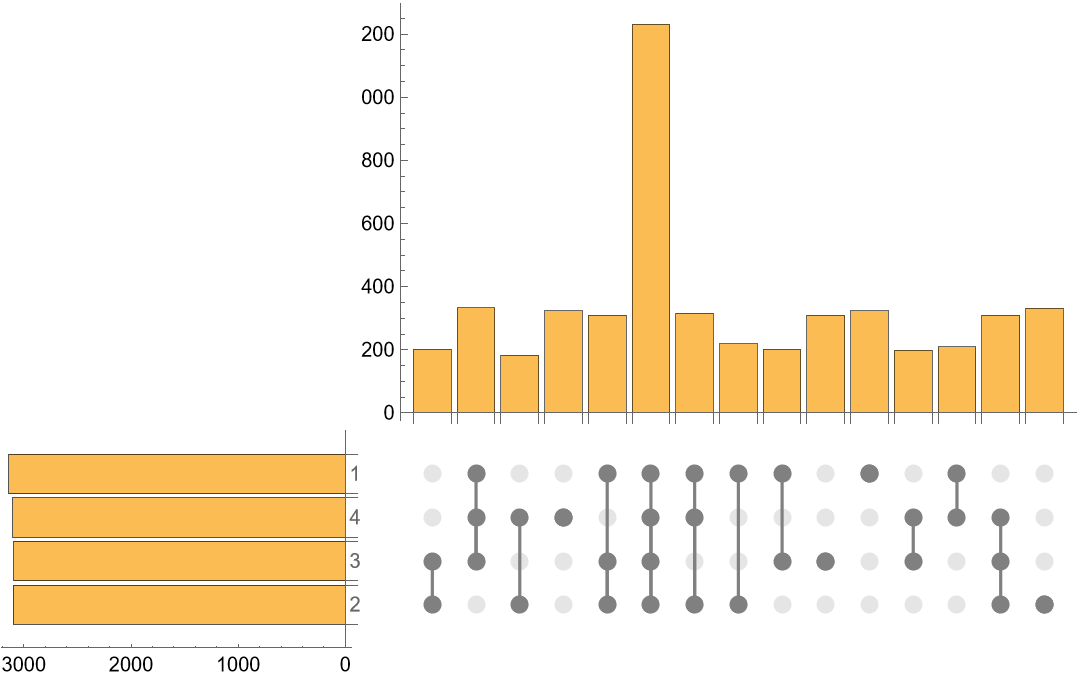Supply ImagePadding specification to display full chart labels:

 In:=Out=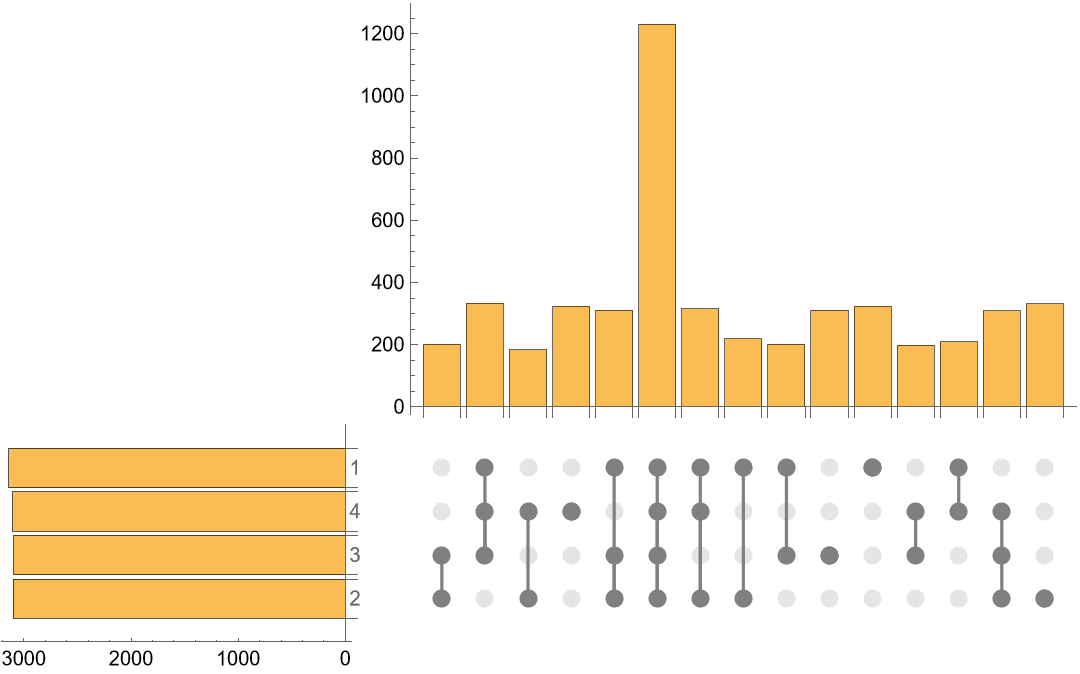ImageSize (3)

Default sizing compresses large combination matrix sub-plots:

 In:=View with default ImageSize:

 In:=Out=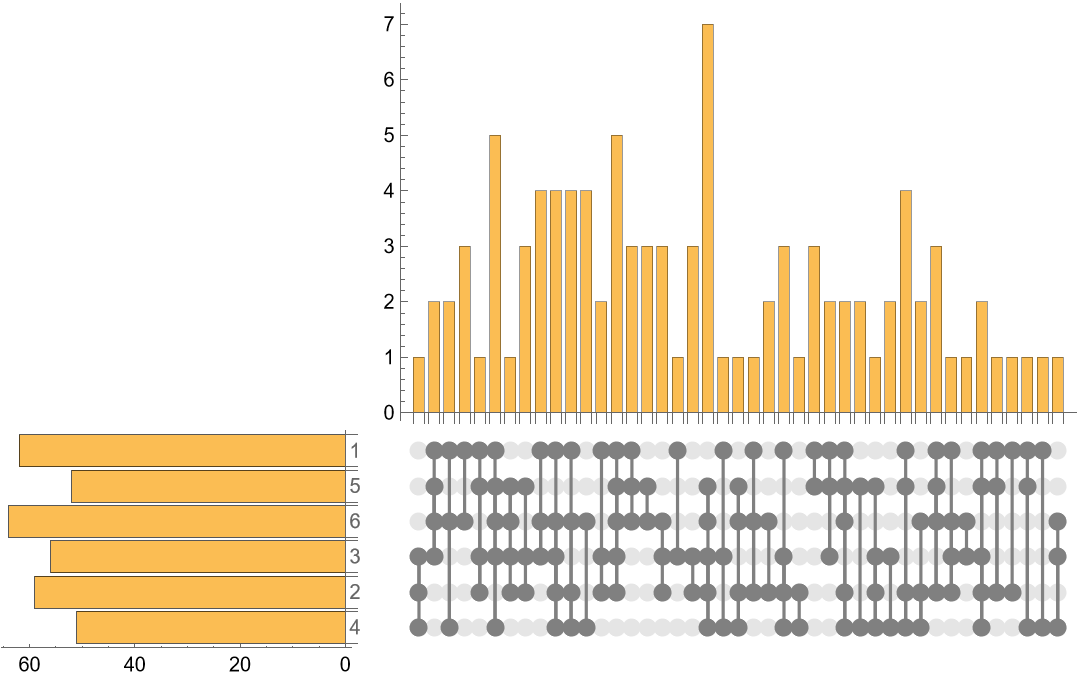Increase ImageSize for larger combination matrix sub-plot:

 In:=Out=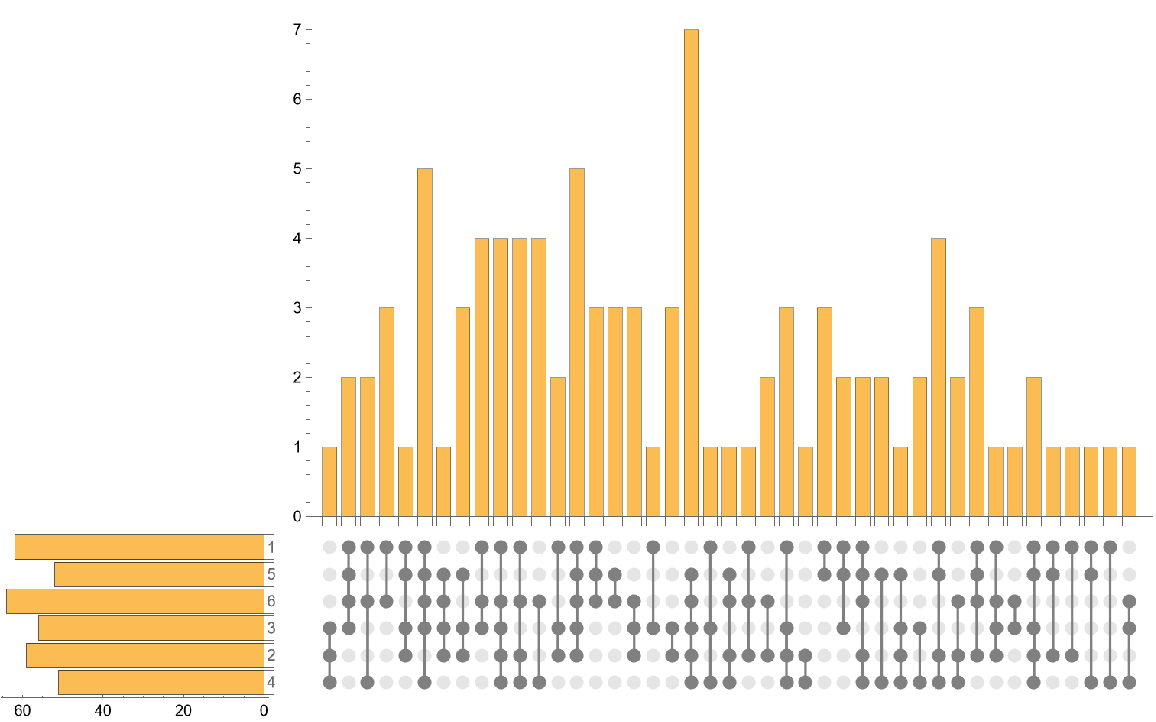PlotMarkers (2)

Create example data:

 In:=Set shape of combination matrix plot markers:

 In:=Out=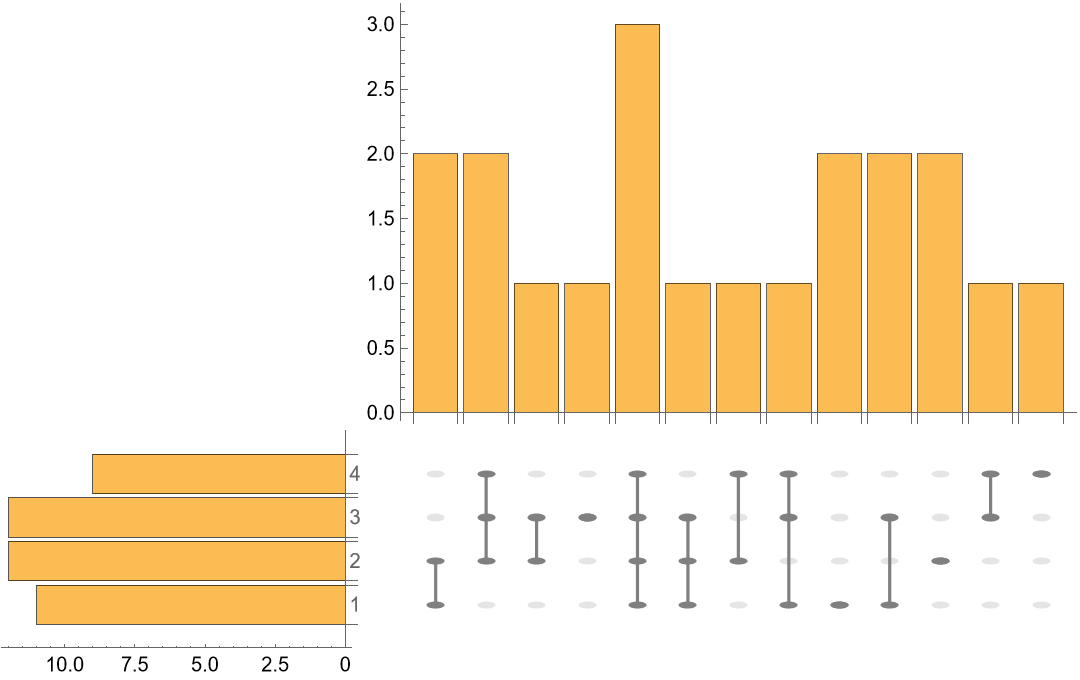PlotStyle (2)

Set PlotStyle of combination matrix plot markers:

 In:=View with PlotStyle specification:

 In:=Out=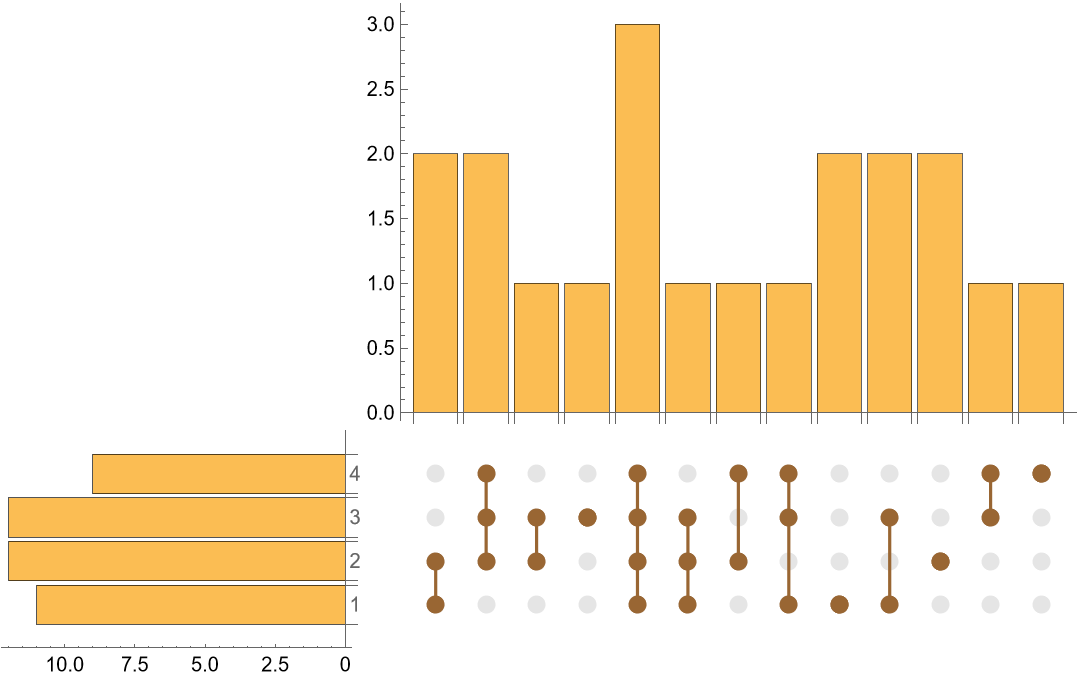PlotTheme (2)

Create example data:

 In:=View with "Monochrome" PlotTheme:

 In:=Out=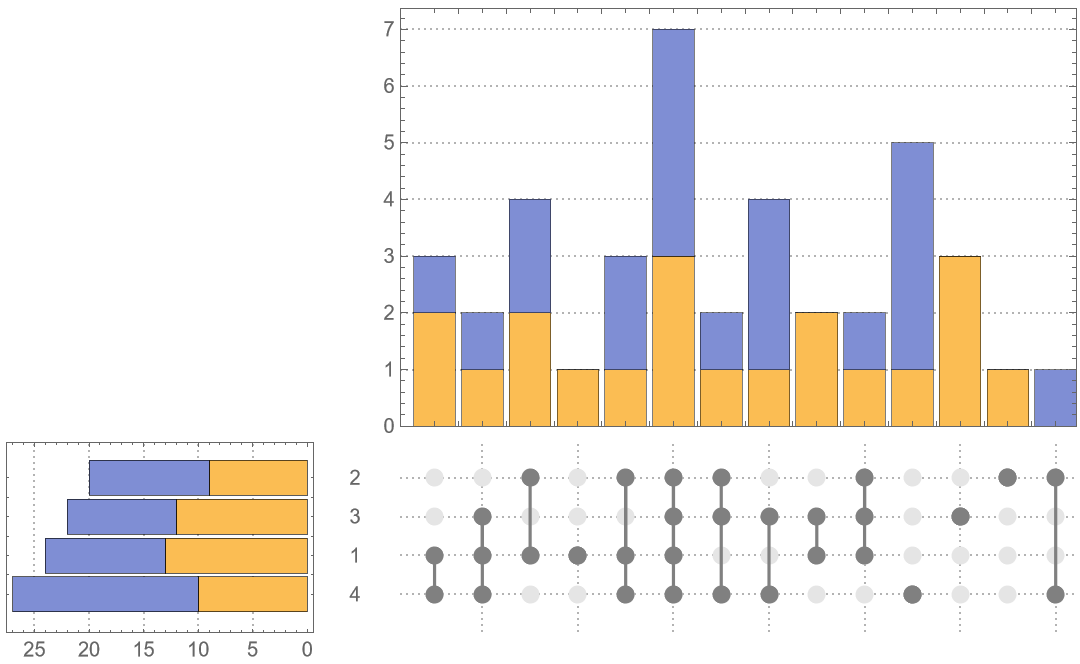SortingFunctions (3)

Create example data:

 In:=Unsorted elements and subsets are listed as they appear in the data:

 In:=Out=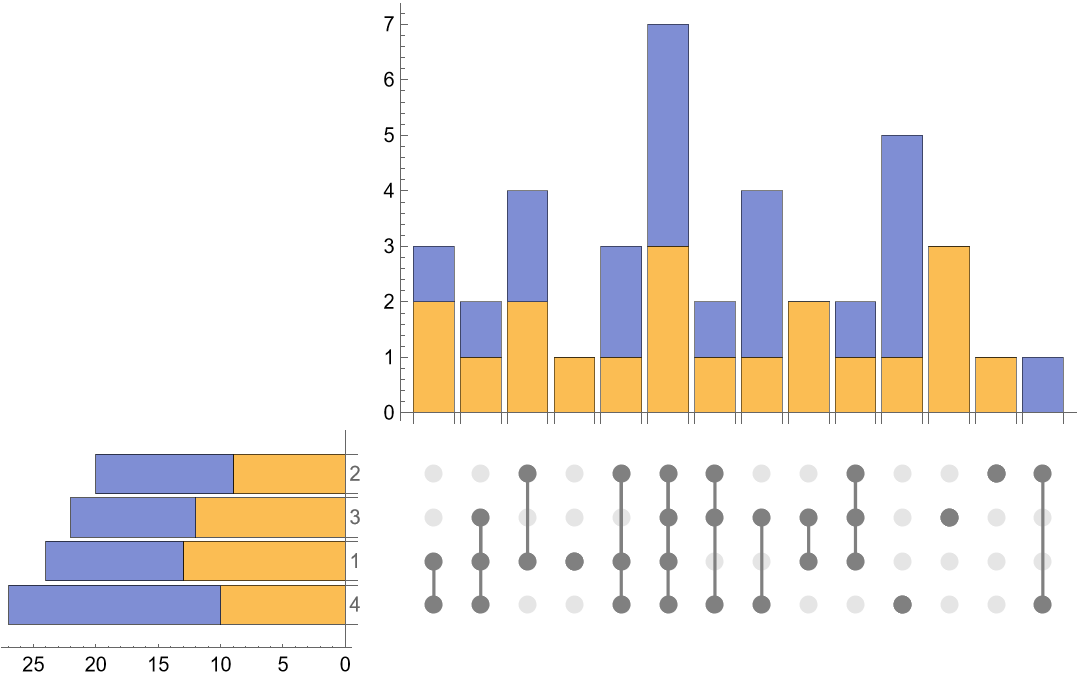Sort set menu sub-chart by its labels and the cardinality sub-chart by the total across all sets in reverse order:

 In:=Out=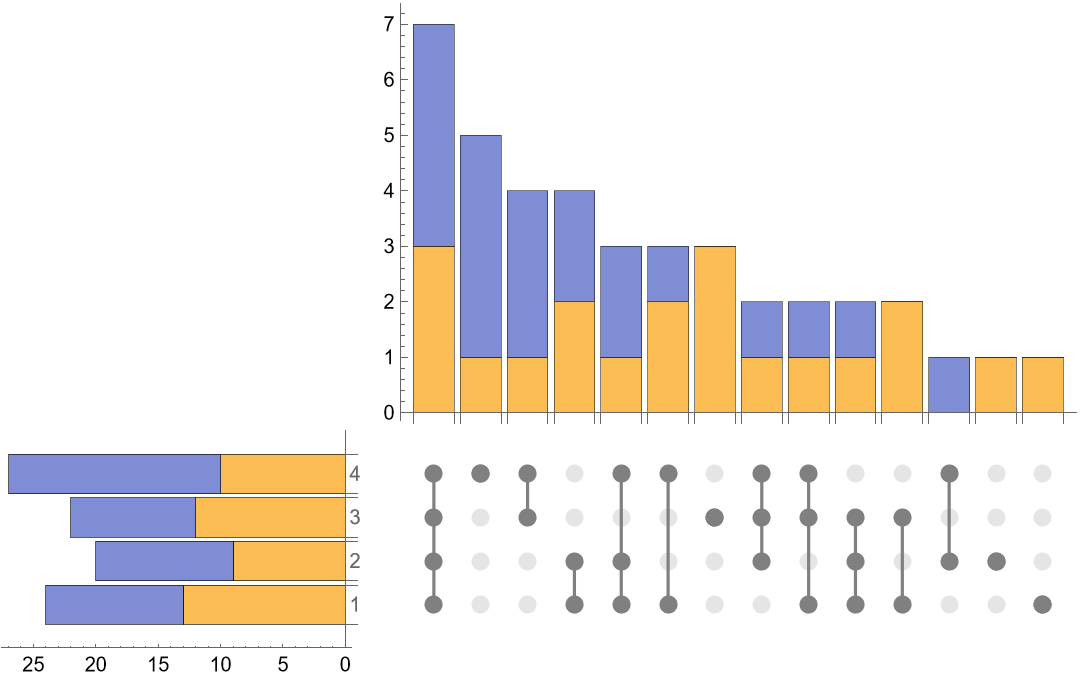Possible Issues (3)

Both the ChartStyle and ChartLegends options must be supplied to return a legend:

 In:=Without both options:

 In:=Out=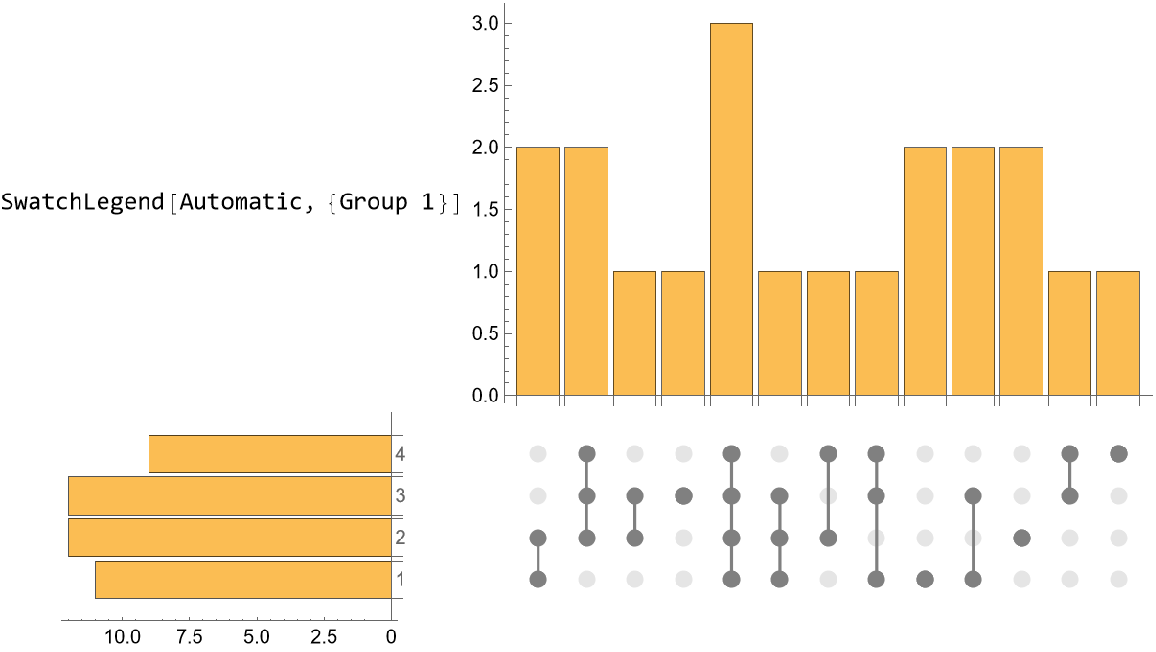With both options:

 In:=Out=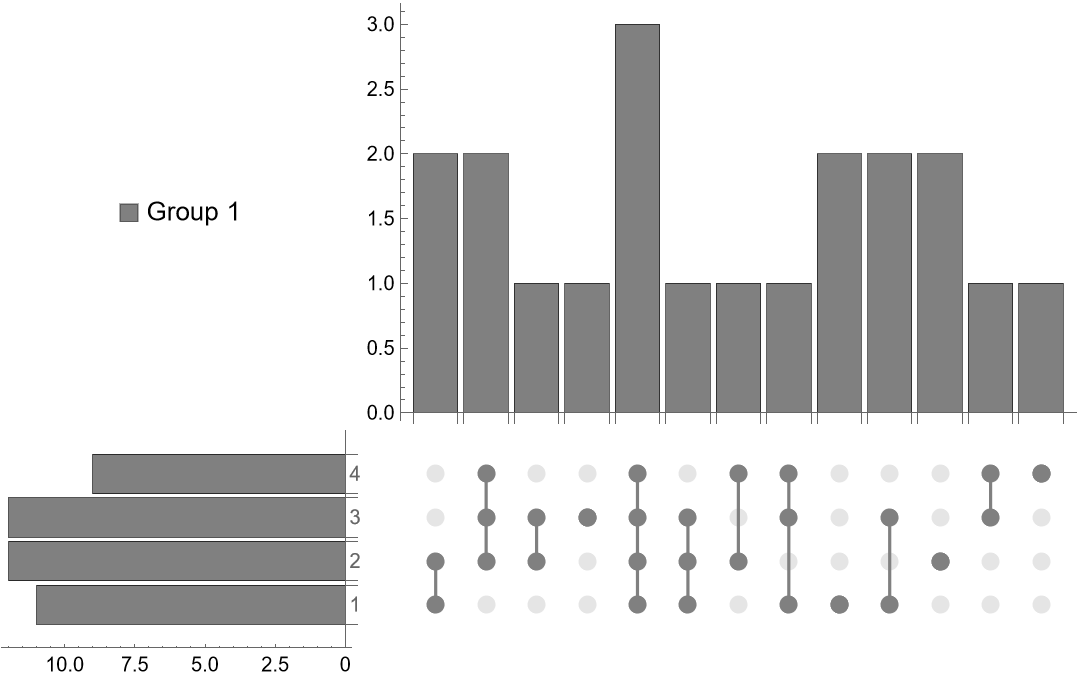Neat Examples (1)

Explore the intersecting sets of class, sex and survived in the Titanic dataset:

 In:=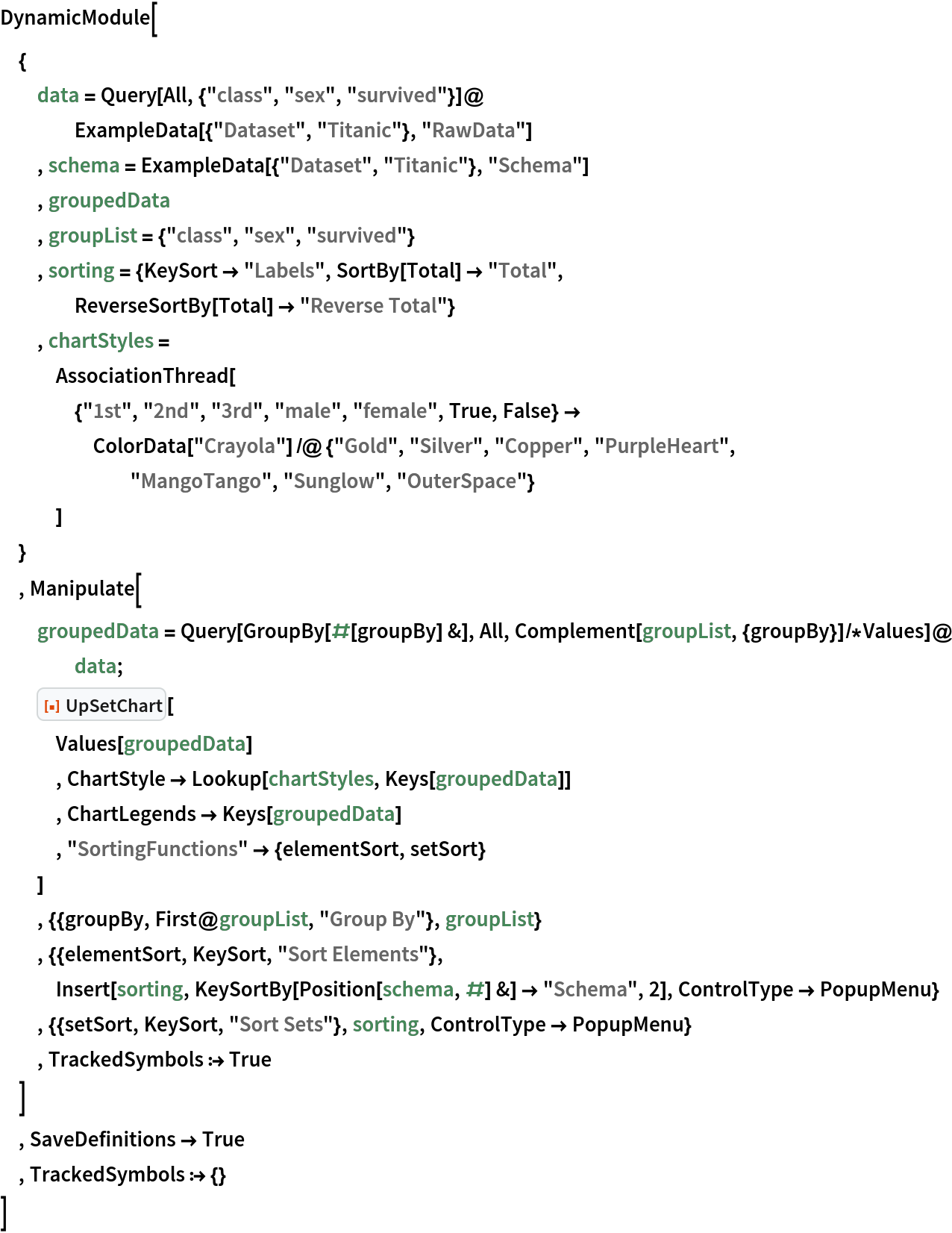Out=